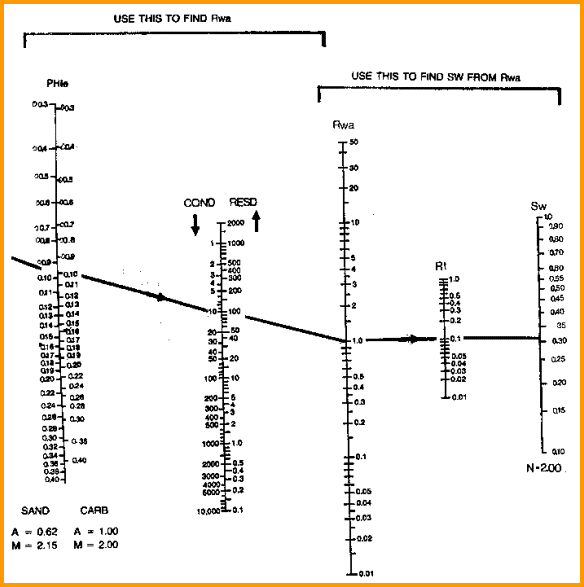Apparent Water Resistivity (Rwa) Method
The Rwa method involves a three step procedure:

STEP 1. Calculate apparent water resistivity for all prospective zones in a well, including water zones:
1: Rwa = (PHIt ^ M) * RESD / A

STEP 2. Select Rw from minimum of Rwa values for all the other defined clean water zones.
2: IF Vsh < 0.2
3: AND IF RESD < RESWET
4: THEN RwaFLAG = 1.0
5: OTHERWISE RwaFLAG = 10 ^ 6
6: RW@FT = min (Rwai * RwaFLAG)

STEP 3. Calculate water saturation from Rwa for each level:
7: Swa = (RW@FT / Rwa) ^ (1 / N)

Where:
A = tortuosity exponent (fractional)
M = cementation exponent (fractional)
N = saturation exponent (fractional)
PHIt = total porosity from any method (fractional)
RESD = resistivity reading on deep log (ohm-m)
RESWET = cut off resistivity for possible water zones (ohm-m)
Rwa = apparent water resistivity (ohm-m)
Rwai = water resistivity of any point in the zone (ohm-m)
RW@FT = formation water resistivity (ohm-m)
Swa = water saturation from Rwa method (fractional)
Vsh = volume of shale (fractional)COMMENTS:
This is merely a restatement of the Archie equation described earlier. The basic Rwa formula is displayed below.

Shale corrected versions of the Rwa equation can be created by rearranging the Simandoux or Dual Water equations. They should be restricted to shaly sands where Vsh < 0.40.RECOMMENDED PARAMETERS:
for carbonates A = 1.00  M = 2.00  N = 2.00  (Archie Equation as first published)
for sandstone  A = 0.62  M = 2.15  N = 2.00  (Humble Equation)
A = 0.81  M = 2.00  N = 2.00  (Tixier Equation - simplified version of Humble Equation)

Asquith (1980 page 67) quoted other authors, giving values for A and M, with N = 2.0, showing the wide range of possible values:
Average sands              A = 1.45  M = 1.54
Shaly sands                  A = 1.65  M = 1.33
Calcareous sands         A = 1.45  M = 1.70
Carbonates                   A = 0.85  M = 2.14
Pliocene sands S.Cal.  A = 2.45  M = 1.08
Miocene LA/TX             A = 1.97  M = 1.29
Clean granular             A = 1.00  M = 2.05 - PHIeNomograph for RWA equation (no shale correction)NUMERICAL EXAMPLE: 1. Using data from Sands A, B, C and D as before: Sand A Sand B Sand C Sand D RESD 20 40 1.2 1.0 PHIe 0.33 0.23 0.30 0.11 A 0.62 0.62 0.62 0.62 M 2.15 2.15 2.15 2.15 Rwa 2.97 2.73 0.145 0.014 RW @ FT 0.9 0.9 0.036 0.015 N 2.0 2.0 2.0 2.0 Swa 0.55 0.57 0.50 1.03

Sample:
Rwa = (0.33 ^ 2.15) * 20 / 0.62 = 2.97
Swa = (0.9 / 2.97) ^ (1 / 2.0) = 0.55

Page Views ---- Since 01 Jan 2015
Copyright 2023 by Accessible Petrophysics Ltd.
CPH Logo, "CPH", "CPH Gold Member", "CPH Platinum Member", "Crain's Rules", "Meta/Log", "Computer-Ready-Math", "Petro/Fusion Scripts" are Trademarks of the Author